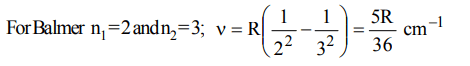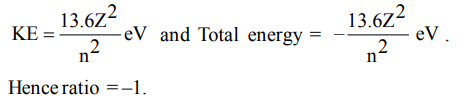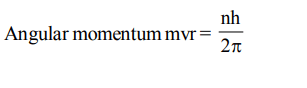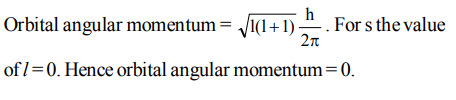## Atomic Structure Questions and Answers Part-6

1. Brackett series are produced when the electrons from the outer orbits jump to
a) 2nd orbit
b) 3rd orbit
c) 4th orbit
d) 5th orbit

Explanation: n1 = 4, n2 = 5, 6, 7 etc.

2. The first emission line in the atomic spectrum of hydrogen in the Balmer series appears at
a) $\frac{9R}{400}cm^{-1}$
b) $\frac{7R}{144}cm^{-1}$
c) $\frac{3R}{4}cm^{-1}$
d) $\frac{5R}{36}cm^{-1}$

Explanation:3. In hydrogen spectrum which of the following lies in the wavelength range 350–700 nm?
a) Balmer series
b) Lyman series
c) Brackett series
d) paschen series

Explanation: 350-700 nm lies in visible region hence Balmer Series

4. In Balmer series of hydrogen atom spectrum which electronic transition causes third line?
a) Fifth Bohr orbit to second one
b) Fifth Bohr orbit to first one
c) Fourth Bohr orbit to second one
d) Fourth Bohr orbit to first one.

Explanation: For Balmer n1 = 2 and n2 = 3, 4, 5. For third line n1 = 2 & n2 = 5.

5. The spectrum of He is expected to be similar to that of
a) H
b) Na
c) $He^{+}$
d) $Li^{+}$

Explanation: Li+ and He both have two electrons each

6. In hydrogen atom, energy of first excited state is –3.4eV. Then find out the KE of the same orbit of hydrogen atom
a) + 3.4 eV
b) + 6.8 eV
c) – 13.6 eV
d) + 13.6 eV

Explanation:7. In the Bohr’s orbit, what is the ratio of total kinetic energy and total energy of the electron?
a) –1
b) –2
c) 1
d) +2

Explanation:8. The kinetic energy of an electron accelerated from rest through a potential difference of 5 V will be
a) 5 J
b) 5 erg
c) 5 eV
d) $8 × 10^{19}eV$

Explanation: 5 eV

9. The angular momentum of an electron revolving around the nucleus is integral multiple of
a) 2n
b) h
c) $h\diagup2\pi$
d) $2\pi n$a) $+\frac{1}{2}.\frac{h}{2\pi}$
c) $\frac{h}{2\pi}$
d) $\sqrt{2} .\frac{h}{2\pi}$